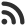Feed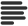Articles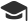Tutorials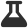CodingLab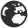CommunityLeaderboardFounder & Programmer at HackersFriend Updated Dec. 26, 2019, 2:40 p.m. ⋅ 45 views

# Remove one element to maximize XOR of array

You are given an array of N integer elements,  let's call it arr.

Your task is to remove 1 element from the array in a way the total XOR value of array is maximized, then print the maximized XOR value of array.

On the first line of input you are given N, total number of elements in array. Then following line will contain N space sperated integers, the elements of array.

Example

Input

``````3
1 1 3``````

Output

``2``

Explanation

All possible deletion and corresponding XOR will look like this:

• Let's remove any of the 1, then  (1 XOR 3) = 2
• Remove 3, then 1 XOR 1) = 0

So, here we see, maximum we can get is 2, so 2 would be the answer output we give.

Solution

Naive approach would be to do it the brute force way. i.e. remove each element one by one and compute the XOR in every case and output the maximum one. But the this is very poor apporach and in most of contests, you'll get timeout error. It's time complexity would be O(N2).

Efficient approach would be this:

• Find the total XOR of entire array and store it in a variable, let's call it, M
• Then, For each element arr[i], perform X = (M XOR arr[i]) and update the final answer as ans = max(X, ans).

Why it works ?

It is simple, it works because if (A XOR B) = C then (C XOR B) = A. Now, to find XOR(arr[0…i-1]) ^ XOR(arr[i+1…N-1]), all we have to perform is XOR(arr) ^ arr[i] where XOR(arr) is the XOR of all the elements of the array.

Implementation

``````// C++ implementation

#include <bits/stdc++.h>
using namespace std;

int maxXOR(int* arr, int n)
{
// Find XOR of the complete array
int xorArr = 0;
for (int i = 0; i < n; i++)
xorArr ^= arr[i];

// To store the final answer
int ans = 0;

// Iterating through the array to find
for (int i = 0; i < n; i++)
ans = max(ans, (xorArr ^ arr[i]));

return ans;
}

// Main function
int main()
{
int n, arr; // keeping it

for(int i=0; i<n; i++)
{
cin>>arr[i];
}

cout << maxXOR(arr, n);

return 0;
}
``````

 arrow_upward UpvoteComment arrow_downward downvote

Events

Nov. 28, 2018, 5:30 p.m.

Python from zero to hero

place Delhi

Aug. 13, 2018, 5:30 p.m.

Python from zero to hero

place Bangalore ( HackersFriend office BTM Layout)